F Distribution and One-Way ANOVA

# 63 Test of Two Variances

This chapter introduces a new probability density function, the F distribution. This distribution is used for many applications including ANOVA and for testing equality across multiple means. We begin with the F distribution and the test of hypothesis of differences in variances. It is often desirable to compare two variances rather than two averages. For instance, college administrators would like two college professors grading exams to have the same variation in their grading. In order for a lid to fit a container, the variation in the lid and the container should be approximately the same. A supermarket might be interested in the variability of check-out times for two checkers. In finance, the variance is a measure of risk and thus an interesting question would be to test the hypothesis that two different investment portfolios have the same variance, the volatility.

In order to perform a F test of two variances, it is important that the following are true:

1. The populations from which the two samples are drawn are approximately normally distributed.
2. The two populations are independent of each other.

Unlike most other hypothesis tests in this book, the F test for equality of two variances is very sensitive to deviations from normality. If the two distributions are not normal, or close, the test can give a biased result for the test statistic.

Suppose we sample randomly from two independent normal populations. Let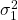and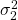be the unknown population variances and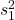and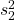be the sample variances. Let the sample sizes be n1 and n2. Since we are interested in comparing the two sample variances, we use the F ratio: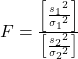F has the distribution F ~ F(n1 – 1, n2 – 1)

where n1 – 1 are the degrees of freedom for the numerator and n2 – 1 are the degrees of freedom for the denominator.

If the null hypothesis is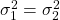, then the F Ratio, test statistic, becomes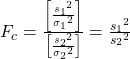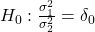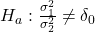if δ0=1 then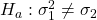Test statistic is :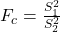The various forms of the hypotheses tested are:

Two-Tailed Test One-Tailed Test One-Tailed Test
H0: σ12 = σ22 H0: σ12 ≤ σ22 H0: σ12 ≥ σ22
H1: σ12 ≠ σ22 H1: σ12 > σ22 H1: σ12 < σ22

A more general form of the null and alternative hypothesis for a two tailed test would be :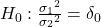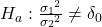Where if δ0 = 1 it is a simple test of the hypothesis that the two variances are equal. This form of the hypothesis does have the benefit of allowing for tests that are more than for simple differences and can accommodate tests for specific differences as we did for differences in means and differences in proportions. This form of the hypothesis also shows the relationship between the F distribution and the χ2 : the F is a ratio of two chi squared distributions a distribution we saw in the last chapter. This is helpful in determining the degrees of freedom of the resultant F distribution.

If the two populations have equal variances, thenandare close in value and the test statistic,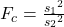is close to one. But if the two population variances are very different,andtend to be very different, too. Choosingas the larger sample variance causes the ratio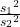to be greater than one. Ifandare far apart, thenis a large number.

Therefore, if F is close to one, the evidence favors the null hypothesis (the two population variances are equal). But if F is much larger than one, then the evidence is against the null hypothesis. In essence, we are asking if the calculated F statistic, test statistic, is significantly different from one.

To determine the critical points we have to find Fα,df1,df2. See Appendix A for the F table. This F table has values for various levels of significance from 0.1 to 0.001 designated as “p” in the first column. To find the critical value choose the desired significance level and follow down and across to find the critical value at the intersection of the two different degrees of freedom. The F distribution has two different degrees of freedom, one associated with the numerator, df1, and one associated with the denominator, df2 and to complicate matters the F distribution is not symmetrical and changes the degree of skewness as the degrees of freedom change. The degrees of freedom in the numerator is n1-1, where n1 is the sample size for group 1, and the degrees of freedom in the denominator is n2-1, where n2 is the sample size for group 2. Fα,df1,df2 will give the critical value on the upper end of the F distribution.

To find the critical value for the lower end of the distribution, reverse the degrees of freedom and divide the F-value from the table into one.

• Upper tail critical value : Fα,df1,df2
• Lower tail critical value : 1/Fα,df2,df1

When the calculated value of F is between the critical values, not in the tail, we cannot reject the null hypothesis that the two variances came from a population with the same variance. If the calculated F-value is in either tail we cannot accept the null hypothesis just as we have been doing for all of the previous tests of hypothesis.

An alternative way of finding the critical values of the F distribution makes the use of the F-table easier. We note in the F-table that all the values of F are greater than one therefore the critical F value for the left hand tail will always be less than one because to find the critical value on the left tail we divide an F value into the number one as shown above. We also note that if the sample variance in the numerator of the test statistic is larger than the sample variance in the denominator, the resulting F value will be greater than one. The shorthand method for this test is thus to be sure that the larger of the two sample variances is placed in the numerator to calculate the test statistic. This will mean that only the right hand tail critical value will have to be found in the F-table.

Two college instructors are interested in whether or not there is any variation in the way they grade math exams. They each grade the same set of 10 exams. The first instructor’s grades have a variance of 52.3. The second instructor’s grades have a variance of 89.9. Test the claim that the first instructor’s variance is smaller. (In most colleges, it is desirable for the variances of exam grades to be nearly the same among instructors.) The level of significance is 10%.

Let 1 and 2 be the subscripts that indicate the first and second instructor, respectively.

n1 = n2 = 10.

H0: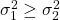and Ha: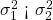Calculate the test statistic: By the null hypothesis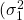, the F statistic is: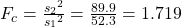Critical value for the test:F9,9 = 5.35 where n1 – 1 = 9 and n2 – 1 = 9.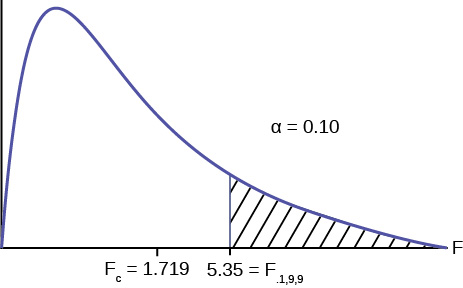Make a decision: Since the calculated F value is not in the tail we cannot reject H0.

Conclusion: With a 10% level of significance, from the data, there is insufficient evidence to conclude that the variance in grades for the first instructor is smaller.

Try It

The New York Choral Society divides male singers up into four categories from highest voices to lowest: Tenor1, Tenor2, Bass1, Bass2. In the table are heights of the men in the Tenor1 and Bass2 groups. One suspects that taller men will have lower voices, and that the variance of height may go up with the lower voices as well. Do we have good evidence that the variance of the heights of singers in each of these two groups (Tenor1 and Bass2) are different?

Tenor1 Bass 2 Tenor 1 Bass 2 Tenor 1 Bass 2
69 72 67 72 68 67
72 75 70 74 67 70
71 67 65 70 64 70
66 75 72 66 69
76 74 70 68 72
74 72 68 75 71
71 72 64 68 74
66 74 73 70 75
68 72 66 72

The histograms are not as normal as one might like. Plot them to verify. However, we proceed with the test in any case.

Subscripts: T1= tenor1 and B2 = bass 2

The standard deviations of the samples are sT1 = 3.3302 and sB2 = 2.7208.

The hypotheses are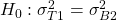and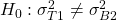(two tailed test)

The F statistic is 1.4894 with 20 and 25 degrees of freedom.

The p-value is 0.3430. If we assume alpha is 0.05, then we cannot reject the null hypothesis.

We have no good evidence from the data that the heights of Tenor1 and Bass2 singers have different variances (despite there being a significant difference in mean heights of about 2.5 inches.)

### References

“MLB Vs. Division Standings – 2012.” Available online at http://espn.go.com/mlb/standings/_/year/2012/type/vs-division/order/true.

### Chapter Review

The F test for the equality of two variances rests heavily on the assumption of normal distributions. The test is unreliable if this assumption is not met. If both distributions are normal, then the ratio of the two sample variances is distributed as an F statistic, with numerator and denominator degrees of freedom that are one less than the samples sizes of the corresponding two groups. A test of two variances hypothesis test determines if two variances are the same. The distribution for the hypothesis test is the F distribution with two different degrees of freedom.

Assumptions:
1. The populations from which the two samples are drawn are normally distributed.
2. The two populations are independent of each other.

Use the following information to answer the next two exercises. There are two assumptions that must be true in order to perform an F test of two variances.

Name one assumption that must be true.

The populations from which the two samples are drawn are normally distributed.

What is the other assumption that must be true?

Use the following information to answer the next five exercises. Two coworkers commute from the same building. They are interested in whether or not there is any variation in the time it takes them to drive to work. They each record their times for 20 commutes. The first worker’s times have a variance of 12.1. The second worker’s times have a variance of 16.9. The first worker thinks that he is more consistent with his commute times. Test the claim at the 10% level. Assume that commute times are normally distributed.

State the null and alternative hypotheses.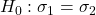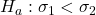or

H0: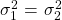Ha: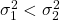What is s1 in this problem?

What is s2 in this problem?

4.11

What is n?

What is the F statistic?

0.7159

What is the critical value?

Is the claim accurate?

No, at the 10% level of significance, we cannot reject the null hypothesis and state that the data do not show that the variation in drive times for the first worker is less than the variation in drive times for the second worker.

Use the following information to answer the next four exercises. Two students are interested in whether or not there is variation in their test scores for math class. There are 15 total math tests they have taken so far. The first student’s grades have a standard deviation of 38.1. The second student’s grades have a standard deviation of 22.5. The second student thinks his scores are more consistent.

State the null and alternative hypotheses.

What is the F Statistic?

2.8674

What is the critical value?

At the 5% significance level, do we reject the null hypothesis?

Cannot accept the null hypothesis. There is enough evidence to say that the variance of the grades for the first student is higher than the variance in the grades for the second student.

Use the following information to answer the next three exercises. Two cyclists are comparing the variances of their overall paces going uphill. Each cyclist records his or her speeds going up 35 hills. The first cyclist has a variance of 23.8 and the second cyclist has a variance of 32.1. The cyclists want to see if their variances are the same or different. Assume that commute times are normally distributed.

State the null and alternative hypotheses.

What is the F Statistic?

0.7414

At the 5% significance level, what can we say about the cyclists’ variances?

### Homework

Three students, Linda, Tuan, and Javier, are given five laboratory rats each for a nutritional experiment. Each rat’s weight is recorded in grams. Linda feeds her rats Formula A, Tuan feeds his rats Formula B, and Javier feeds his rats Formula C. At the end of a specified time period, each rat is weighed again and the net gain in grams is recorded.

Linda’s rats Tuan’s rats Javier’s rats
43.5 47.0 51.2
39.4 40.5 40.9
41.3 38.9 37.9
46.0 46.3 45.0
38.2 44.2 48.6

Determine whether or not the variance in weight gain is statistically the same among Javier’s and Linda’s rats. Test at a significance level of 10%.

1.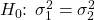2.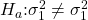3. df(num) = 4; df(denom) = 4
4. F4, 4
5. 3.00
6. Check student’t solution.
7. Decision: Cannot reject the null hypothesis; Conclusion: There is insufficient evidence to conclude that the variances are different.

A grassroots group opposed to a proposed increase in the gas tax claimed that the increase would hurt working-class people the most, since they commute the farthest to work. Suppose that the group randomly surveyed 24 individuals and asked them their daily one-way commuting mileage. The results are as follows.

Working-class Professional (middle incomes) Professional (wealthy)
17.8 16.5 8.5
26.7 17.4 6.3
49.4 22.0 4.6
9.4 7.4 12.6
65.4 9.4 11.0
47.1 2.1 28.6
19.5 6.4 15.4
51.2 13.9 9.3

Determine whether or not the variance in mileage driven is statistically the same among the working class and professional (middle income) groups. Use a 5% significance level.

Use the following information to answer the next two exercises. The following table lists the number of pages in four different types of magazines.

Home decorating News Health Computer
172 87 82 104
286 94 153 136
163 123 87 98
205 106 103 207
197 101 96 146

Which two magazine types do you think have the same variance in length?

Which two magazine types do you think have different variances in length?

The answers may vary. Sample answer: Home decorating magazines and news magazines have different variances.

Is the variance for the amount of money, in dollars, that shoppers spend on Saturdays at the mall the same as the variance for the amount of money that shoppers spend on Sundays at the mall? Suppose that the (Figure) shows the results of a study.

Saturday Sunday Saturday Sunday
75 44 62 137
18 58 0 82
150 61 124 39
94 19 50 127
62 99 31 141
73 60 118 73
89

Are the variances for incomes on the East Coast and the West Coast the same? Suppose that (Figure) shows the results of a study. Income is shown in thousands of dollars. Assume that both distributions are normal. Use a level of significance of 0.05.

East West
38 71
47 126
30 42
82 51
75 44
52 90
115 88
67
1. H0: ==2. Ha:3. df(n) = 7, df(d) = 6
4. F7,6
5. 0.8117
6. 0.7825
7. Check student’s solution.
1. Alpha: 0.05
2. Decision: Cannot reject the null hypothesis.
3. Reason for decision: calculated test statistics is not in the tail of the distribution
4. Conclusion: There is not sufficient evidence to conclude that the variances are different.

Thirty men in college were taught a method of finger tapping. They were randomly assigned to three groups of ten, with each receiving one of three doses of caffeine: 0 mg, 100 mg, 200 mg. This is approximately the amount in no, one, or two cups of coffee. Two hours after ingesting the caffeine, the men had the rate of finger tapping per minute recorded. The experiment was double blind, so neither the recorders nor the students knew which group they were in. Does caffeine affect the rate of tapping, and if so how?

Here are the data:

0 mg 100 mg 200 mg 0 mg 100 mg 200 mg
242 248 246 245 246 248
244 245 250 248 247 252
247 248 248 248 250 250
242 247 246 244 246 248
246 243 245 242 244 250

King Manuel I, Komnenus ruled the Byzantine Empire from Constantinople (Istanbul) during the years 1145 to 1180 A.D. The empire was very powerful during his reign, but declined significantly afterwards. Coins minted during his era were found in Cyprus, an island in the eastern Mediterranean Sea. Nine coins were from his first coinage, seven from the second, four from the third, and seven from a fourth. These spanned most of his reign. We have data on the silver content of the coins:

First coinage Second coinage Third coinage Fourth coinage
5.9 6.9 4.9 5.3
6.8 9.0 5.5 5.6
6.4 6.6 4.6 5.5
7.0 8.1 4.5 5.1
6.6 9.3 6.2
7.7 9.2 5.8
7.2 8.6 5.8
6.9
6.2

Did the silver content of the coins change over the course of Manuel’s reign?

Here are the means and variances of each coinage. The data are unbalanced.

First Second Third Fourth
Mean 6.7444 8.2429 4.875 5.6143
Variance 0.2953 1.2095 0.2025 0.1314

Here is a strip chart of the silver content of the coins: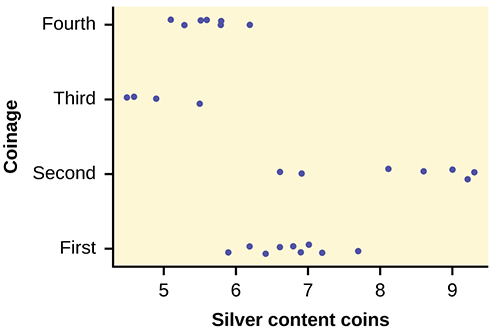While there are differences in spread, it is not unreasonable to use ANOVA techniques. Here is the completed ANOVA table:

Source of variation Sum of squares (SS) Degrees of freedom (df) Mean square (MS) F
Factor (Between) 37.748 4 – 1 = 3 12.5825 26.272
Error (Within) 11.015 27 – 4 = 23 0.4789
Total 48.763 27 – 1 = 26

P(F > 26.272) = 0;

Cannot accept the null hypothesis for any alpha. There is sufficient evidence to conclude that the mean silver content among the four coinages are different. From the strip chart, it appears that the first and second coinages had higher silver contents than the third and fourth.

The American League and the National League of Major League Baseball are each divided into three divisions: East, Central, and West. Many years, fans talk about some divisions being stronger (having better teams) than other divisions. This may have consequences for the postseason. For instance, in 2012 Tampa Bay won 90 games and did not play in the postseason, while Detroit won only 88 and did play in the postseason. This may have been an oddity, but is there good evidence that in the 2012 season, the American League divisions were significantly different in overall records? Use the following data to test whether the mean number of wins per team in the three American League divisions were the same or not. Note that the data are not balanced, as two divisions had five teams, while one had only four.

Division Team Wins
East NY Yankees 95
East Baltimore 93
East Tampa Bay 90
East Toronto 73
East Boston 69
Division Team Wins
Central Detroit 88
Central Chicago Sox 85
Central Kansas City 72
Central Cleveland 68
Central Minnesota 66
Division Team Wins
West Oakland 94
West Texas 93
West LA Angels 89
West Seattle 75

Here is a stripchart of the number of wins for the 14 teams in the AL for the 2012 season.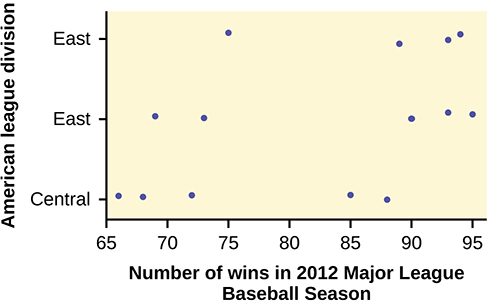While the spread seems similar, there may be some question about the normality of the data, given the wide gaps in the middle near the 0.500 mark of 82 games (teams play 162 games each season in MLB). However, one-way ANOVA is robust.

Here is the ANOVA table for the data:

Source of variation Sum of squares (SS) Degrees of freedom (df) Mean square (MS) F
Factor (Between) 344.16 3 – 1 = 2 172.08
Error (Within) 1,219.55 14 – 3 = 11 110.87 1.5521
Total 1,563.71 14 – 1 = 13

P(F > 1.5521) = 0.2548
Since the p-value is so large, there is not good evidence against the null hypothesis of equal means. We cannot reject the null hypothesis. Thus, for 2012, there is not any have any good evidence of a significant difference in mean number of wins between the divisions of the American League.